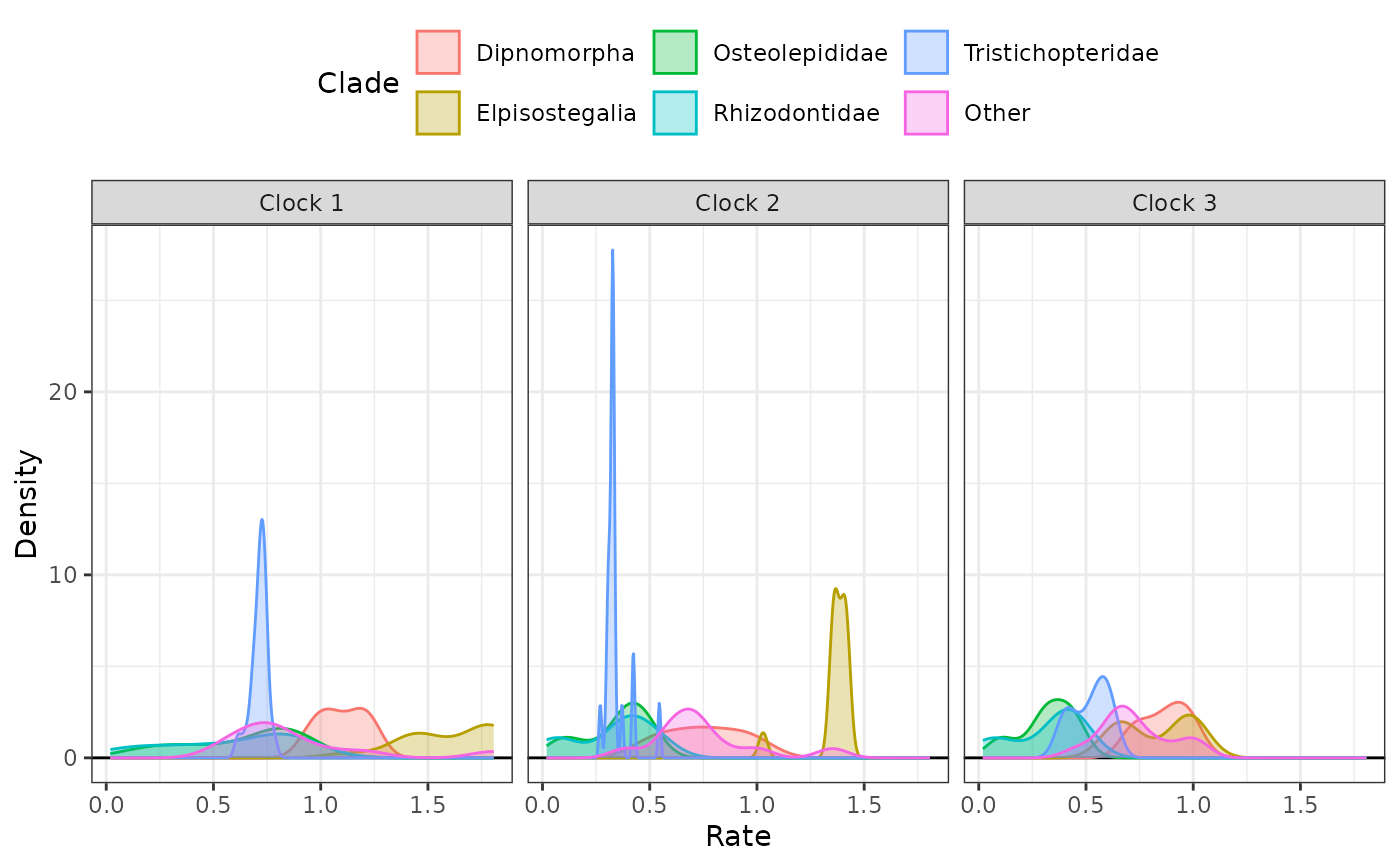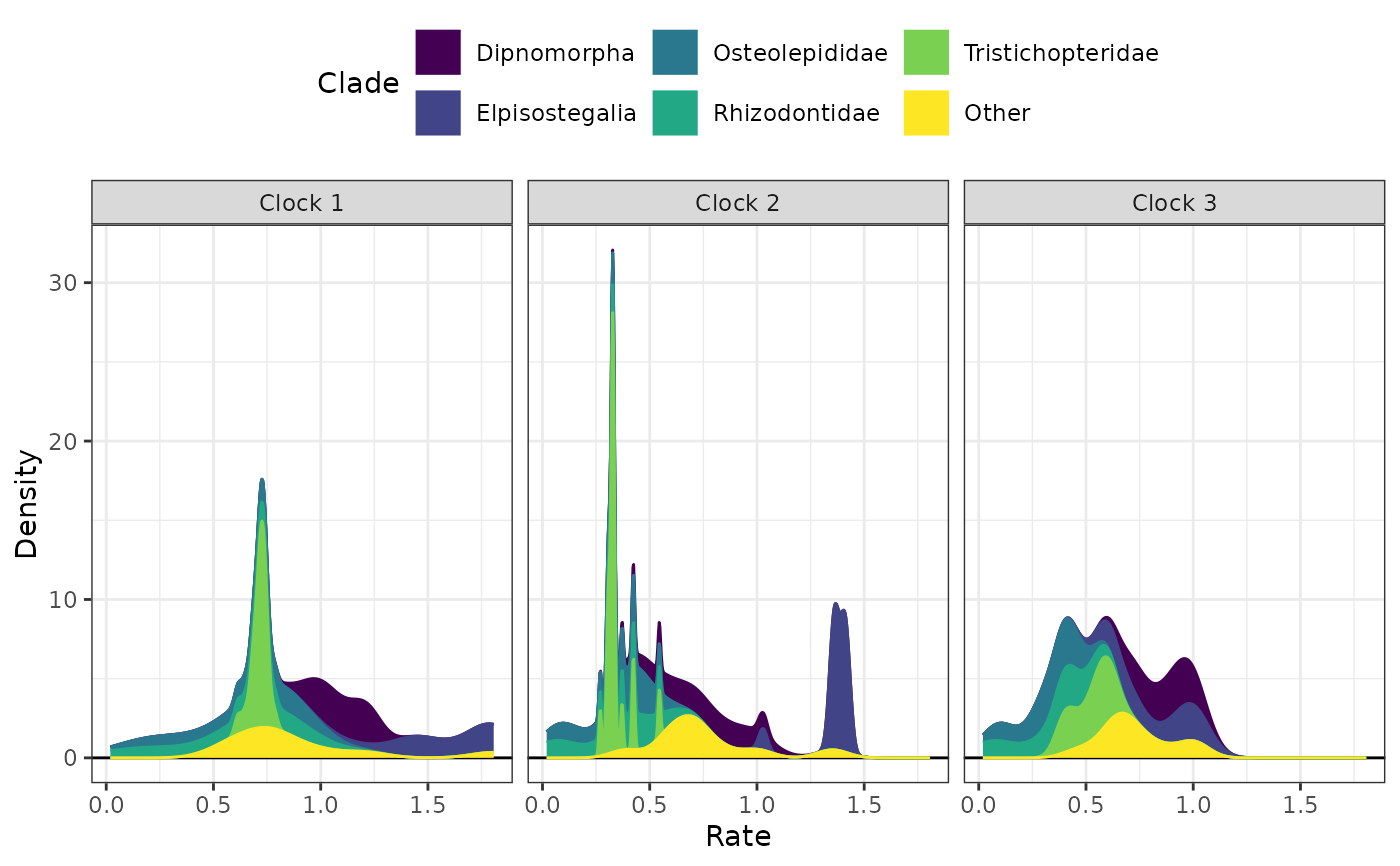Plots the distribution density of clock rates by clock and clade. The input must have a "clade" column.

## Usage

clockrate_dens_plot(rate_table, clock = NULL,
stack = FALSE, nrow = 1,
scales = "fixed")

## Arguments

rate_table

A data frame of clock rates, such as from the output of get_clockrate_table_MrBayes with an extra "clade" column.

clock

Which clock rates will be plotted. If unspecified, all clocks are plotted.

stack

Whether to display stacked density plots (TRUE) or overlapping density plots (FALSE).

nrow

When plotting rates for more than one clock, how many rows should be filled by the plots. This is passed to facet_wrap.

scales

When plotting rates for more than one clock, whether the axis scales should be "fixed" (default) across clocks or allowed to vary ("free", "free_x", or "free_y"). This is passed to facet_wrap.

## Details

The user must manually add clades to the rate table produced by get_clockrate_table_MrBayes before it can be used with this function. This can be doen manually with in R, such as by using a graphical user interface for editing data like the DataEditR package, or by writing the rate table to a spreadsheet and reading it back in after adding the clades. The example below uses a table that has had the clades added.

## Value

A ggplot object, which can be modified using ggplot2 functions.

vignette("rates-selection") for the use of this function as part of an analysis pipeline.

get_clockrate_table_MrBayes, geom_density

## Examples

# See vignette("rates-selection") for how to use this
# function as part of an analysis pipeline

# Overlapping plots